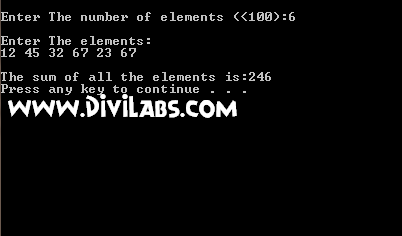# Write A C++ Program (WAP) To Find The Sum Of All The Elements In An Integer Array

/* The following C++ program will 1st ask the user to enter the number of elements in the array, which should be less than 100. Yes, you can increase this number to even more by just increasing the value under the declaration a to whichever the number you want, e.g, a. Then the user is asked about the array element & then the program will calculate the sum of all the elements & display the sum of all the element*/

#include <iostream>
using namespace std;
//using standard namespace

int main ()
{
int a,n,t=0,i;
//initialising t with 0 in order to avoid any garbage value to occur
cout<<"\nEnter The number of elements (<100):";
cin>>n;
cout<<"\nEnter The elements:\n";
for(i=0;i<n;i++)
cin>>a[i];
//inputting the elements in the array
for(i=0;i<n;i++)
t=t+a[i];
//calculating the sum of array elements
cout<<"\nThe sum of all the elements is:"<<t<<endl;
system("pause");
return (0);
}

Sample input/output: